# Winch

The steel rope has a diameter of 6mm and a length of 20m.
We are winding on drum width 60mm, starting diameter 50mm. What is the final diameter after winding?

Correct result:

D2 =  138 mm

#### Solution:

$D_{1}=50 \ \text{mm} \ \\ l_{1}=60 \ \text{mm} \ \\ d=6 \ \text{mm} \ \\ l_{2}=20 \ m \rightarrow mm=20 \cdot \ 1000 \ mm=20000 \ mm \ \\ \ \\ r_{1}=D_{1}/2=50/2=25 \ \text{mm} \ \\ \ \\ V_{1}=\pi \cdot \ r_{1}^2 \cdot \ l_{1}=3.1416 \cdot \ 25^2 \cdot \ 60 \doteq 117809.7245 \ \text{mm}^3 \ \\ V_{2}=d^2 \cdot \ l_{2} + V_{1}=6^2 \cdot \ 20000 + 117809.7245 \doteq 837809.7245 \ \text{mm}^3 \ \\ \ \\ V_{2}=\pi \cdot \ r_{2}^2 \cdot \ l_{1} \ \\ \ \\ r_{2}=\sqrt{ \dfrac{ V_{2} }{ \pi \cdot \ l_{1} } }=\sqrt{ \dfrac{ 837809.7245 }{ 3.1416 \cdot \ 60 } } \doteq 66.6687 \ \text{mm} \ \\ \ \\ D_{3}=2 \cdot \ r_{2}=2 \cdot \ 66.6687 \doteq 133.3374 \ \text{mm} \ \\ D_{2}=d \cdot \ \lceil D_{3}/d \rceil=6 \cdot \ \lceil 133.3374/6 \rceil=138 \ \text{mm}$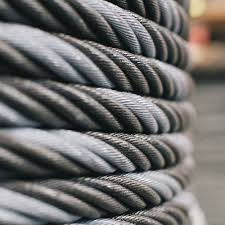Our examples were largely sent or created by pupils and students themselves. Therefore, we would be pleased if you could send us any errors you found, spelling mistakes, or rephasing the example. Thank you!

Please write to us with your comment on the math problem or ask something. Thank you for helping each other - students, teachers, parents, and problem authors.Tips to related online calculators
Do you want to convert length units?
Do you know the volume and unit volume, and want to convert volume units?

## Next similar math problems:

• Gas sphere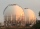The gas tank has the shape of a sphere with a diameter of 14 m. How many m3 of gas will fit in it?
• Volume ratio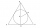Calculate the volume ratio of balls circumscribed (diameter r) and inscribed (diameter ϱ) into an equilateral rotating cone.
• Cylinder containerThe cylindrical container with a diameter of 1.8 m contains 2,000 liters of water. How high does the water reach?
• The trench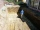Calculate how many cubic meters of soil needs to be removed from the excavation in the shape of an isosceles trapezoid, the top width is 3 meters, the lower width is 1.8 m, the depth of the excavation is 1 m, and the length is 20 m.
• Truncated cone 6Calculate the volume of the truncated cone whose bases consist of an inscribed circle and a circle circumscribed to the opposite sides of the cube with the edge length a=1.
• Gas, lpg and diesel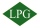Calculate how much it costs to drive 35000 km by car: a) a car with a consumption of 8l/100 km of LPG for 60 cents b) 7l/100 km of petrol for 1.33 euros c) 6 l/100 km of diesel for 1.2 euros. Calculate what is cheaper.
• Four prismsQuestion No. 1: The prism has the dimensions a = 2.5 cm, b = 100 mm, c = 12 cm. What is its volume? a) 3000 cm2 b) 300 cm2 c) 3000 cm3 d) 300 cm3 Question No.2: The base of the prism is a rhombus with a side length of 30 cm and a height of 27 cm. The heig
• Clay balls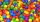How many clay balls with a radius of 1 cm can be made from a ball of clay with a radius of 8 cm?
• Mixing 5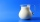Carlos mixed 4/15 of chocolate syrup with 1/2 of milk. Determine the reasonable estimate of the total amount of liquid
• Mixing paint with waterMr. Adamek will paint. The purchased paint is diluted with water in a ratio of 1: 1.5. a) how many parts of water will add to 1 part of the paint b) how many liters of water the mission adds to 2 liters of paint
• Flower boxesHow many m2 of 10mm thick boards are needed to make 12 flower boxes? The dimensions of the box are 180,150 and 1500 mm.
• Equilateral coneWe pour so much water into a container that has the shape of an equilateral cone, the base of which has a radius r = 6 cm, that one-third of the volume of the cone is filled. How high will the water reach if we turn the cone upside down?Calculate the volume of a quadrilateral pyramid, the base of which has the shape of a rectangle with dimensions a = 6cm, b = 4cm and height v = 11cm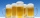When checking compliance with the beer tapping, it was found that 60% of the offered beers were underfilled. The others were fine. Thus, instead of 0.5 l, the volume was 4.4 dcl on average. What was the volume of one average underfilled beer?Find the volume of a regular hexagonal pyramid, the base edge of which is 12 cm long and the side edge 20 cm.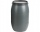In the backyard is an empty barrel with a volume of 2 hl. How many liters are missing at the edge, when 53 liters flowed into it for 1 day and 68 liters of water for 2 days?The cylinder has a shell content of 300 cm square, while the height of the cylinder is 12 cm. Calculate the volume of this cylinder.